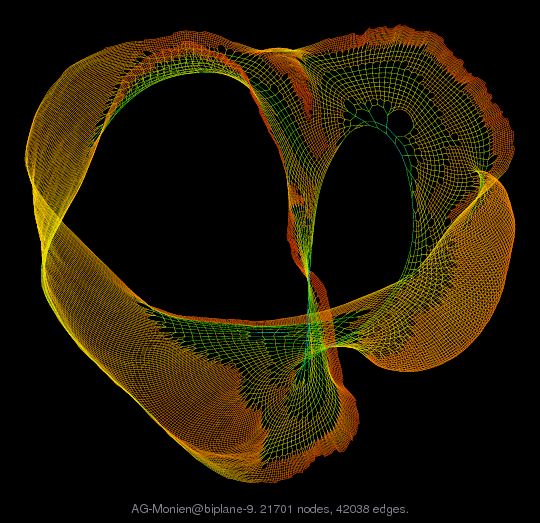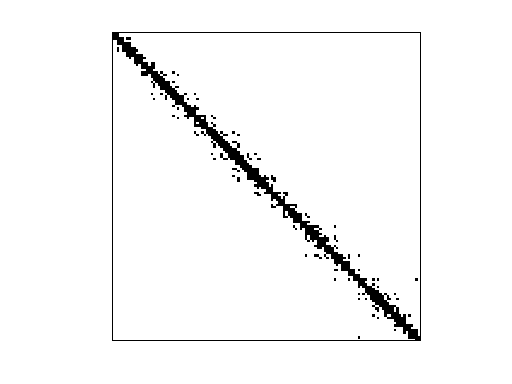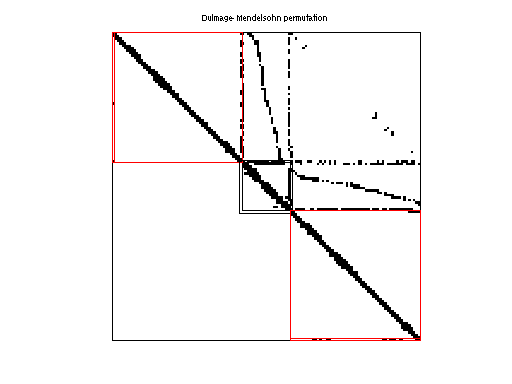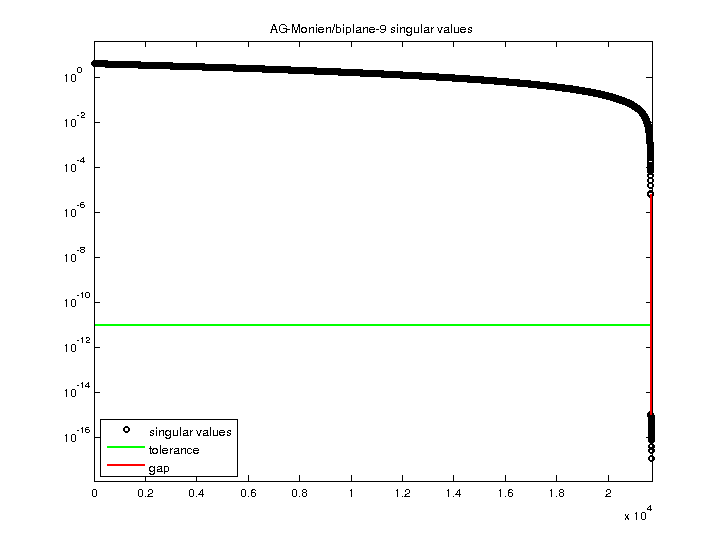Matrix: AG-Monien/biplane-9

Description: 2D grid(undirected graph drawing)• Matrix group: AG-Monien
• download as a MATLAB mat-file, file size: 167 KB. Use UFget(2433) or UFget('AG-Monien/biplane-9') in MATLAB.

 Matrix properties number of rows 21,701 number of columns 21,701 nonzeros 84,076 structural full rank? no structural rank 21,691 # of blocks from dmperm 277 # strongly connected comp. 1 explicit zero entries 0 nonzero pattern symmetry symmetric numeric value symmetry symmetric type binary structure symmetric Cholesky candidate? no positive definite? no

 author R. Diekmann, R. Preis editor R. Diekmann, R. Preis date 1998 kind 2D/3D problem 2D/3D problem? yes

 Additional fields size and type coord full 21701-by-2

Notes:

```AG-Monien Graph Collection, Ralf Diekmann and Robert Preis

A collection of test graphs from various sources.  Many of the graphs
include XY or XYZ coordinates.  This set also includes some graphs from
the Harwell-Boeing collection, the NASA matrices, and some random matrices
which are not included here in the AG-Monien/ group of the UF Collection.

AG-Monien/big : same as Nasa/barth5, Pothen/barth5 (not included here)
AG-Monien/cage_3_11 : same as Pajek/GD98_c (included here)

The AG-Monien/GRID subset is not included.  It contains square grids that
are already well-represented in the UF Collection.

Six of the problem sets are included as sequences, each sequence being
a single problem instance in the UF Collection:

bfly:  10 butterfly graphs 3..12
cage:  45 cage graphs 3..12
cca:   10 cube-connected cycle graphs, no wrap
ccc:   10 cube-connected cycle graphs, with wrap
debr:  18 De Bruijn graphs
se:    13 shuffle-exchange graphs

Problem.aux.G{:} are the graphs in these 6 sequences.  Problem.aux.Gname{:}
are the original names of each graph, and Problemm.aux.Gcoord{:} are the
xy or xyz coordinates of each node, if present.
```

 Ordering statistics: result nnz(chol(P*(A+A'+s*I)*P')) with AMD 342,013 Cholesky flop count 1.5e+07 nnz(L+U), no partial pivoting, with AMD 662,325 nnz(V) for QR, upper bound nnz(L) for LU, with COLAMD 409,961 nnz(R) for QR, upper bound nnz(U) for LU, with COLAMD 758,576

 SVD-based statistics: norm(A) 3.99612 min(svd(A)) 1.14588e-17 cond(A) 3.48738e+17 rank(A) 21,659 sprank(A)-rank(A) 32 null space dimension 42 full numerical rank? no singular value gap 5.71582e+09

 singular values (MAT file): click here SVD method used: s = svd (full (R)) ; where [~,R,E] = spqr (A) with droptol of zero status: ok